NR Interference Modeling with Toroidal Wrap-Around

This example models a 19-cell cluster with toroidal wrap-around as described in ITU-R M.2101-0. The wrap-around provides uniform interference at the cluster edge. All the cells in the cluster operate in the same frequency band with the serving gNB at the center of the cell. You can enable or disable the wrap-around to observe that with wrap-around, the performance metrics of an edge cell becomes similar to the center cell.

Introduction

The example considers these operations within the gNB and user equipment (UE).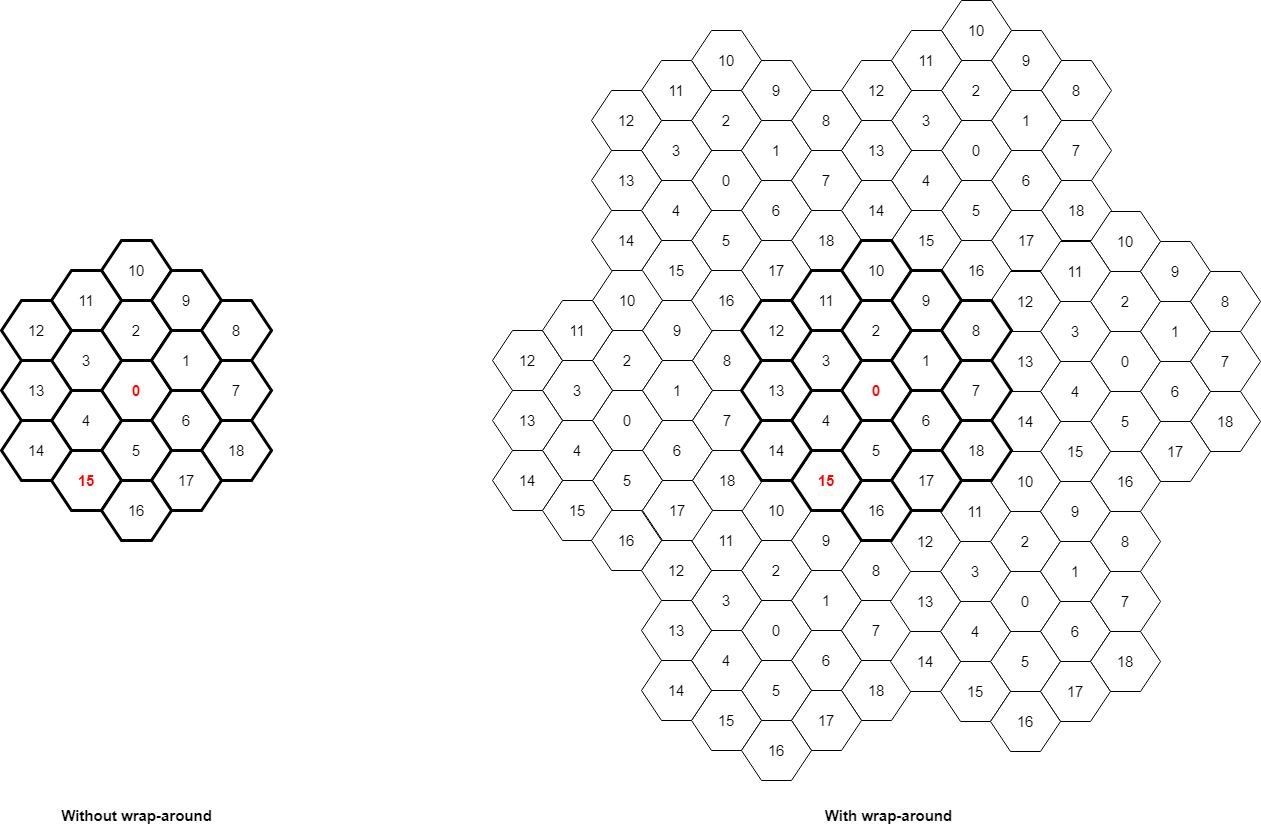This example models:

• Co-channel intercell interference with wrap-around modeling for removing edge effects

• PUSCH demodulation reference signal (DM-RS) and PDSCH DM-RS

• DL channel quality measurement by UEs based on the CSI-RS received from gNB.

• Single-input single-output (SISO) configuration with omnidirectional antennas.

• Free space path loss (FSPL), and additive white Gaussian noise (AWGN).

The example assumes that control packets such as UL assignment, DL assignment, buffer status report (BSR), PDSCH feedback, and channel quality indicator (CQI) report, are sent out of band, that is, without the need of resources for transmission and assured error-free reception.

Toroidal Wrap-around Modeling

To simulate the behavior of a cellular network without introducing edge effects, this example models an infinite cellular network by using toroidal wrap-around. This figure shows the network region of 19 cells. In the absence of wrap-around, cell 0 of the central cluster, shown in red, is uniformly surrounded and experiences interference from all sides. An edge cell like cell-15 experiences comparatively lesser interference. The wrap-around repeats the original cluster six times to uniformly surround the central cluster.

The entire network region relevant for simulations is a cluster of 19 cells (shown in bold).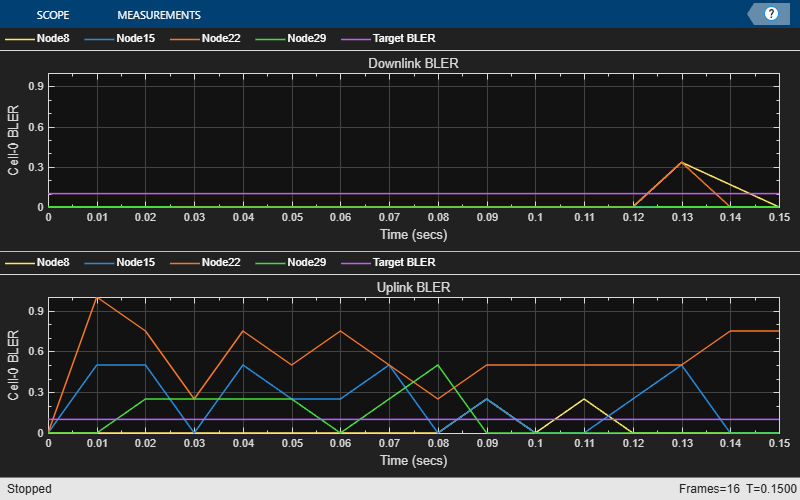In the wrap-around model, the signal or interference from any UE to a cell is treated as if that UE is in the original cell cluster and the gNB in any of the seven clusters as specified in ITU-R M.2101-0. The distances used to compute the path loss from a transmitter node at $\left(a,b\right)$ to a receiver node at $\left(x,y\right)$ is the minimum of these seven distances.

• Distance between $\left(x,y\right)$ and $\left(a,b\right)$

• Distance between $\left(x,y\right)$ and $\left(a-\sqrt{3}D,b-4D\right)$, where $D$ is the distance between two adjacent gNBs (inter-site distance)

• Distance between $\left(x,y\right)$ and $\left(a+\sqrt{3}D,b+4D\right)$

• Distance between $\left(x,y\right)$ and $\left(a-\frac{\sqrt{3}}{2}D,b+\frac{7}{2}D\right)$

• Distance between $\left(x,y\right)$ and $\left(a+\frac{3\sqrt{3}}{2}D,b-\frac{7}{2}D\right)$

• Distance between $\left(x,y\right)$ and $\left(a-\frac{5\sqrt{3}}{2}D,b-\frac{1}{2}D\right)$

• Distance between $\left(x,y\right)$ and $\left(a-\frac{5\sqrt{3}}{2}D,b-\frac{1}{2}D\right)$

To calculate the modified distances between nodes due to the wrap-around, use the hNRNodeDistanceCalculator helper. If you disable wrap-around, then the distance between nodes is the normal Euclidean distance.

NR Protocol Stack

A node (gNB or UE) consists of applications generating traffic, a radio link control (RLC) layer, a medium access control (MAC) layer, and a physical layer (PHY). The hNRGNB and hNRUE helper classes create gNB and UE nodes, respectively, containing RLC, MAC, and PHY layers. For more details on each layer, refer to the 'NR Protocol Stack' section in the NR Cell Performance Evaluation with Physical Layer Integration example.

Scenario Configuration

Configure simulation parameters in the simParameters structure.

rng('default'); % Reset the random number generator
simParameters = []; % Clear the simParameters variable
simParameters.NumFramesSim = 3; % Simulation time, in number of 10 ms frames
simParameters.EnableWrapAround = true; % Enable wrap-around modeling
simParameters.SchedulingType = 0; % Slot-based scheduling

Specify the number of UEs in each cell, assuming that UEs have sequential radio network temporary identifiers (RNTIs) from 1 to simParameters.NumUEs. If you change the number of UEs, you must ensure that these simulation parameters are arrays of length equal to the value of simParameters.NumUEs: simParameters.UEDistance, ulAppDataRate, dlAppDataRate.

simParameters.NumUEs = 2; % Number of UEs in each cell
simParameters.UEDistance = [150; 250]; % Distance of UEs from gNB in meters
simParameters.InterSiteDistance = 1732; % Distance between adjacent gNBs in meters

Set the channel bandwidth to 5 MHz and the subcarrier spacing (SCS) to 15 kHz as defined in section 5.3.2 of 3GPP TS 38.104.

simParameters.NumRBs = 25;
simParameters.SCS = 15; % kHz
simParameters.DLCarrierFreq = 2.635e9; % Hz
simParameters.ULCarrierFreq = 2.515e9; % Hz

Assume that the UL and DL carriers have the same channel bandwidth, and specify the transmit power and antenna gain.

simParameters.DLBandwidth = 5e6; % Hz
simParameters.ULBandwidth = 5e6; % Hz
simParameters.UETxPower = 23; % Tx power for all the UEs in dBm
simParameters.GNBTxPower = 32; % Tx power for gNBs in dBm
simParameters.GNBRxGain = 8; % Receiver antenna gain at gNB in dBi

Specify the signal-to-interference-plus-noise ratio (SINR) to CQI mapping table for a block error rate (BLER) of 0.1.

simParameters.SINR90pc = [-5.46 -0.46 4.54 9.05 11.54 14.04 15.54 18.04 ...
20.04 22.43 24.93 25.43 27.43 30.43 33.43];

Specify the scheduling strategy and the maximum limit on the RBs allotted for PUSCH and PDSCH. The transmission limit applies only to new PUSCH and PDSCH assignments, and not to the retransmissions.

simParameters.SchedulerStrategy = 'PF'; % Supported scheduling strategies: 'PF', 'RR', and 'BestCQI'
simParameters.RBAllocationLimitUL = 20; % For PUSCH
simParameters.RBAllocationLimitDL = 20; % For PDSCH

Logging and visualization configuration

Specify the ID of cell of interest as an integer between 1 and 18, inclusive. The example shows visualizations and metrics for this cell and the central cell, cell-0.

simParameters.CellOfInterest = 15; % Set a value from 1 to 18. Set the value to 0 to visualize cell-0 only.
validateattributes(simParameters.CellOfInterest, {'numeric'}, {'integer', 'scalar', '>=', 0, '<=', 18}, 'simParameters.CellOfInterest', 'CellOfInterest')

The CQIVisualization and RBVisualization parameters control the display of the CQI visualization and the RB assignment visualization respectively. To enable the RB visualization plot, set the RBVisualization field to true.

simParameters.CQIVisualization = true;
simParameters.RBVisualization = false;

Set the enableTraces as true to log the traces. If the enableTraces is set to false, then CQIVisualization and RBVisualization are disabled automatically and traces are not logged in the simulation. To speed up the simulation, set the enableTraces to false.

enableTraces = true;

The example updates the metrics plots periodically. Set the number of updates during the simulation.

simParameters.NumMetricsSteps = 3;

Write the logs to MAT-files. The example uses these logs for post-simulation analysis and visualization.

parametersLogFile = 'simParameters'; % For logging the simulation parameters
simulationLogFile = 'simulationLogs'; % For logging the simulation traces
simulationMetricsFile = 'simulationMetrics'; % For logging the simulation metrics

Application traffic configuration

Set the periodic UL and DL application traffic pattern for UEs.

ulAppDataRate = [16e3; 16e3]; % UL application data rate in kilo bits per second (kbps)
dlAppDataRate = [16e3; 16e3]; % DL application data rate in kbps

Derived Parameters

Compute the derived parameters based on the primary configuration parameters specified in the previous section, and set some example-specific constants.

simParameters.DuplexMode = 0; % Frequency-division duplexing (FDD)
numSitesPerCluster = 19; % Number of gNBs per cluster

Set the UE and gNB positions.

simParameters.GNBPositions = hMacrocellTopology(simParameters);
simParameters.UEPositions = cell(numSitesPerCluster, 1);
for idx = 1:numSitesPerCluster
theta = rand(simParameters.NumUEs, 1)*(2*pi);
% Set the UE position with respect to the gNB location
simParameters.UEPositions{idx} = simParameters.GNBPositions(idx, :) + simParameters.UEDistance.*[cos(theta) sin(theta) zeros(simParameters.NumUEs, 1)];
end
simParameters.NCellIDList = 0:numSitesPerCluster-1; % List of physical cell IDs

Specify the CSI-RS resource configuration, assuming that all UEs measure channel quality on the same CSI-RS resource.

csirsConfig = cell(1, numSitesPerCluster);
for cellIdx = 1:numSitesPerCluster
% Possible row numbers for single transmit antenna case are 1 and 2
csirsConfig{cellIdx} = nrCSIRSConfig('NID', simParameters.NCellIDList(cellIdx), 'NumRB', simParameters.NumRBs, 'RowNumber', 2, 'SubcarrierLocations', 1, 'SymbolLocations', 0);
end
csiReportConfig = struct('SubbandSize', 8, 'CQIMode', 'Subband');
simParameters.CSIReportConfig = {csiReportConfig};

Calculate the slot duration for the selected SCS and the number of slots in a 10 ms frame.

slotDuration = 1/(simParameters.SCS/15); % In ms
numSlotsFrame = 10/slotDuration; % Number of slots in a 10 ms frame
numSlotsSim = simParameters.NumFramesSim*numSlotsFrame; % Number of slots in the simulation

Set the interval at which the example updates metrics visualization, number of slots. Because this example uses a time granularity of one slot, the MetricsStepSize field must be an integer.

simParameters.MetricsStepSize = ceil(numSlotsSim / simParameters.NumMetricsSteps);
if mod(numSlotsSim, simParameters.NumMetricsSteps) ~= 0
% Update the NumMetricsSteps parameter if it does not exactly divide NumSlotsSim
simParameters.NumMetricsSteps = floor(numSlotsSim / simParameters.MetricsStepSize);
end

Specify one logical channel in each UE, and set the logical channel configuration for all nodes (UEs and gNBs) in the example.

numLogicalChannels = 1;
simParameters.LCHConfig.LCID = 4; % Logical channel ID (logical channel ID of data radio bearers starts from 4)

Specify the RLC entity type in the range [0, 3]. The values 0, 1, 2, and 3 indicate RLC UM unidirectional DL entity, RLC UM unidirectional UL entity, RLC UM bidirectional entity, and RLC AM entity, respectively.

simParameters.RLCConfig.EntityType = 2;

Create RLC channel configuration structure.

rlcChannelConfigStruct.LCGID = 1; % Mapping between logical channel and logical channel group ID
rlcChannelConfigStruct.Priority = 1; % Priority of each logical channel
rlcChannelConfigStruct.PBR = 8; % Prioritized bitrate (PBR), in kilobytes per second, of each logical channel
rlcChannelConfigStruct.BSD = 10; % Bucket size duration (BSD), in ms, of each logical channel
rlcChannelConfigStruct.EntityType = simParameters.RLCConfig.EntityType;
rlcChannelConfigStruct.LogicalChannelID = simParameters.LCHConfig.LCID;

Set the maximum RLC service data unit (SDU) length, in bytes, as specified in 3GPP TS 38.323, and the total number of nodes in the simulation.

maxRLCSDULength = 9000;
simParameters.MaxReceivers = numSitesPerCluster*(simParameters.NumUEs + 1); % Number of nodes
if ~isfield(simParameters, 'SchedulingType') || simParameters.SchedulingType == 0 % No scheduling type or slot-based scheduling
tickGranularity = 14;
simParameters.PUSCHMappingType = 'A';
simParameters.PDSCHMappingType = 'A';
else % Symbol-based scheduling
tickGranularity = 1;
simParameters.PUSCHMappingType = 'B';
simParameters.PDSCHMappingType = 'B';
end

Multicell Setup

Set up the 19-cell topology with each cell consisting of one gNB and one UE. For each cell, create the gNB and UE objects, initialize the channel quality information for UEs, and set up the logical channel at gNB and UEs. The hNRGNB and hNRUE helper classes create gNB and UE nodes respectively, containing the RLC, MAC, and PHY layers.

gNB = cell(numSitesPerCluster, 1);
UEs = cell(numSitesPerCluster, simParameters.NumUEs);

Create DL and UL packet distribution objects, and initialize a wrap-around distance calculator object.

% Create packet distribution object
packetDistributionObj = hNRPacketDistribution(simParameters);
distCalcFcn = hNRNodeDistanceCalculator(simParameters.InterSiteDistance); % Function handle to calculate wrap-around distances
cellParam = simParameters;
for siteIdx = 1:numSitesPerCluster
cellParam.NCellID = simParameters.NCellIDList(siteIdx); % Cell ID
cellParam.Position = simParameters.GNBPositions(siteIdx, :);
cellParam.CSIRSConfig = csirsConfig(siteIdx);
% Create scheduler
switch(simParameters.SchedulerStrategy)
case 'RR' % Round robin scheduler
scheduler = hNRSchedulerRoundRobin(cellParam);
case 'PF' % Proportional fair scheduler
scheduler = hNRSchedulerProportionalFair(cellParam);
case 'BestCQI' % Best CQI scheduler
scheduler = hNRSchedulerBestCQI(cellParam);
end

% Create gNB
gNB{siteIdx} = hNRGNB(cellParam);
gNB{siteIdx}.PhyEntity = hNRGNBPhy(cellParam); % Create PHY layer instance
configurePhy(gNB{siteIdx}, cellParam); % Configure PHY layer
if simParameters.EnableWrapAround
% Register distance calculator at gNB for wrap-around distance computations
gNB{siteIdx}.DistanceCalculatorFcn = distCalcFcn;
end
setPhyInterface(gNB{siteIdx}); % Set up the interface to PHY layer

ueParam = cellParam;
for ueIdx = 1:simParameters.NumUEs
ueParam.Position = simParameters.UEPositions{siteIdx}(ueIdx, :); % Position of UE in (x,y,z) coordinates
ueParam.CSIReportConfig = csiReportConfig;
UEs{siteIdx, ueIdx} = hNRUE(ueParam, ueIdx);
UEs{siteIdx, ueIdx}.PhyEntity = hNRUEPhy(ueParam, ueIdx); % Create PHY layer instance
configurePhy(UEs{siteIdx, ueIdx}, ueParam); % Configure PHY layer
if simParameters.EnableWrapAround
% Register distance calculator at the UEs for wrap-around distance computations
UEs{siteIdx, ueIdx}.DistanceCalculatorFcn = distCalcFcn;
end
setPhyInterface(UEs{siteIdx, ueIdx}); % Set up the interface to PHY

% Set up logical channel at gNB for the UE
configureLogicalChannel(gNB{siteIdx}, ueIdx, rlcChannelConfigStruct);
% Set up logical channel at UE
configureLogicalChannel(UEs{siteIdx, ueIdx}, ueIdx, rlcChannelConfigStruct);

% Add data traffic pattern generators to gNB and UE nodes
% Create an object for On-Off network traffic pattern and add it to the
% specified UE. This object generates the uplink data traffic on the UE
ulApp = networkTrafficOnOff('PacketSize', maxRLCSDULength, 'GeneratePacket', true, ...
'OnTime', simParameters.NumFramesSim/100, 'OffTime', 0, 'DataRate', ulAppDataRate(ueIdx));

% Create an object for On-Off network traffic pattern for the specified
% UE and add it to the gNB. This object generates the downlink data
% traffic on the gNB for the UE
dlApp = networkTrafficOnOff('PacketSize', maxRLCSDULength, 'GeneratePacket', true, ...
'OnTime', simParameters.NumFramesSim/100, 'OffTime', 0, 'DataRate', dlAppDataRate(ueIdx));
end
% Setup the UL and DL packet distribution mechanism
hNRSetUpPacketDistribution(cellParam, gNB{siteIdx}, UEs(siteIdx, :), packetDistributionObj);
end

Set up logging and visualization, specifying the central cell (cell 0) and the cell of interest.

cellsOfInterest = unique([0; simParameters.CellOfInterest]);
numCellsOfInterest = length(cellsOfInterest); % Number of cells that the example logs and visualizes

Visualize the network topology

hTopologyVisualizer(simParameters);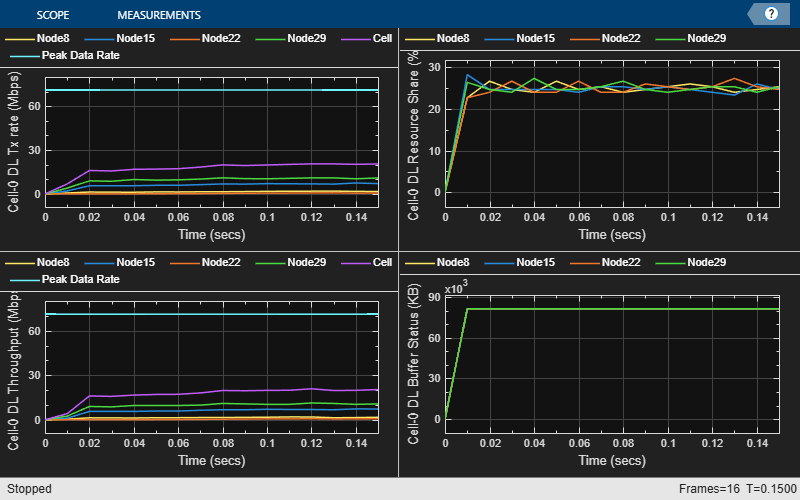Log and visualize MAC and PHY metrics and traces.

simSchedulingLogger = cell(numCellsOfInterest, 1);
simPhyLogger = cell(numCellsOfInterest, 1);
metricsVisualizer = cell(numCellsOfInterest, 1);

for siteIdx = 1:numCellsOfInterest
simParameters.NCellID = cellsOfInterest(siteIdx);
if enableTraces
% Create an object for MAC scheduling traces logging
simSchedulingLogger{siteIdx} = hNRSchedulingLogger(simParameters);
% Create an object for PHY layer traces logging
simPhyLogger{siteIdx} = hNRPhyLogger(simParameters);

if simParameters.CQIVisualization || simParameters.RBVisualization
gridVisualizer = hNRGridVisualizer(simParameters, 'CellOfInterest', simParameters.NCellID, 'MACLogger', simSchedulingLogger{siteIdx});
end
end

% Create visualization object for MAC and PHY metrics
nodes = struct('UEs', {UEs(simParameters.NCellID+1, :)}, 'GNB', gNB{simParameters.NCellID+1});
metricsVisualizer{siteIdx} = hNRMetricsVisualizer(simParameters, 'Nodes', nodes, 'CellOfInterest', simParameters.NCellID, ...
'EnableSchedulerMetricsPlots', true, 'EnablePhyMetricsPlots', true);
end

Processing Loop

Simulation is run slot by slot. For each cell, in each slot, these operations are executed:

• Run the MAC and PHY layers of gNB

• Run the MAC and PHY layers of UEs

• Log and visualize metrics for each layer

• Advance the timer for the nodes and send a trigger to application and RLC layers every millisecond. The application layer and RLC layer execute their scheduled operations based on a 1 ms timer trigger.

slotNum = 0;
numSymbolsSim = numSlotsSim * 14; % Simulation time in units of symbol duration (assuming normal cyclic prefix)

% Run processing loop
for symbolNum = 1 : tickGranularity : numSymbolsSim
if mod(symbolNum - 1, 14) == 0
slotNum = slotNum + 1;
end
% Because all the cells operate on the same SCS, slot durations do not vary
for siteIdx = 1:numSitesPerCluster % For each site
run(gNB{siteIdx});

% Run MAC and PHY layers of UEs
for ueIdx = 1:simParameters.NumUEs
run(UEs{siteIdx, ueIdx});
end

if enableTraces
cellIdx = find((siteIdx-1) == cellsOfInterest, 1);
if ~isempty(cellIdx)
% MAC logging
logCellSchedulingStats(simSchedulingLogger{cellIdx}, symbolNum, gNB{siteIdx}, UEs(siteIdx, :));

% PHY logging
logCellPhyStats(simPhyLogger{cellIdx}, symbolNum, gNB{siteIdx}, UEs(siteIdx, :));
end
end
end

for siteIdx = 1:numSitesPerCluster
% Advance timer ticks for gNB and UEs by the number of symbols per slot
for ueIdx = 1:simParameters.NumUEs
end
end

% Visualization
for idx = 1:numCellsOfInterest
% Check slot boundary
if symbolNum > 1 && ((simParameters.SchedulingType == 1 && mod(symbolNum, 14) == 0) || (simParameters.SchedulingType == 0 && mod(symbolNum-1, 14) == 0))
% If the update periodicity is reached, plot scheduler metrics and PHY metrics at slot boundary
if mod(slotNum, simParameters.MetricsStepSize) == 0
plotLiveMetrics(metricsVisualizer{idx});
end
end
end
end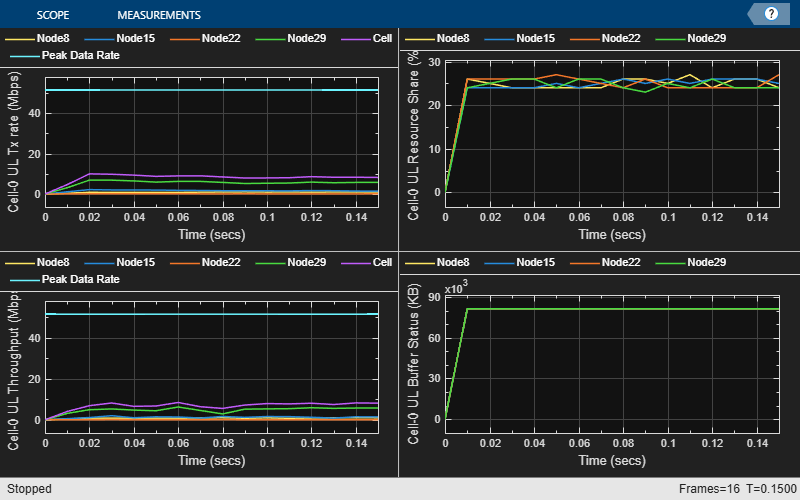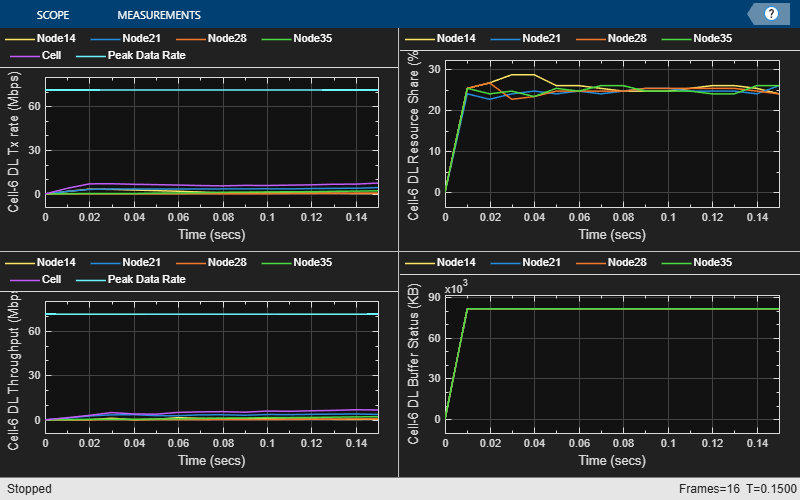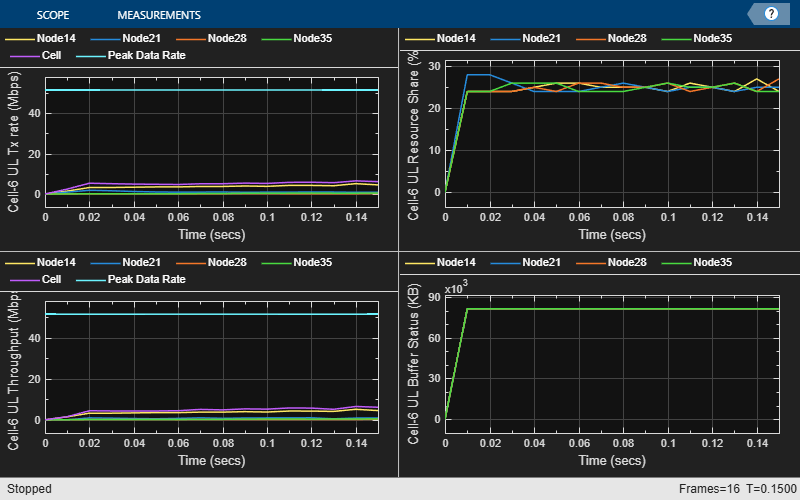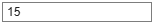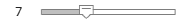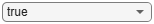Get the simulation metrics of the simParameters.CellOfInterest and save it in a MAT-file. The simulation metrics are saved in a MAT-file with the file name as simulationMetricsFile. At the end of the simulation, the achieved value for system performance indicators is compared to their theoretical peak values (considering zero overheads). Performance indicators displayed are achieved data rate (UL and DL), achieved spectral efficiency (UL and DL), and BLER observed for UEs (DL and UL). The peak values are calculated as per 3GPP TR 37.910.

metricsList = cell(numCellsOfInterest, 1);
metricStruct = struct('NCellID',[], 'Metrics',[]);
for siteIdx = 1:numCellsOfInterest
metricStruct.NCellID = cellsOfInterest(siteIdx);
metricStruct.Metrics = getMetrics(metricsVisualizer{siteIdx});
metricsList{siteIdx} = metricStruct;
% Display the performance indicators
fprintf('\n\nMetrics for cell %d :\n\n', metricStruct.NCellID);
displayPerformanceIndicators(metricsVisualizer{siteIdx})
end
Metrics for cell 0 :
Peak UL Throughput: 31.11 Mbps. Achieved Cell UL Throughput: 3.20 Mbps
Achieved UL Throughput for each UE: [2.05        1.15]
Achieved Cell UL Goodput: 3.20 Mbps
Achieved UL Goodput for each UE: [2.05        1.15]
Peak UL spectral efficiency: 6.22 bits/s/Hz. Achieved UL spectral efficiency for cell: 0.64 bits/s/Hz

Peak DL Throughput: 31.11 Mbps. Achieved Cell DL Throughput: 4.35 Mbps
Achieved DL Throughput for each UE: [2.64        1.71]
Achieved Cell DL Goodput: 3.94 Mbps
Achieved DL Goodput for each UE: [2.57        1.37]
Peak DL spectral efficiency: 6.22 bits/s/Hz. Achieved DL spectral efficiency for cell: 0.79 bits/s/Hz

Block error rate for each UE in the uplink direction: [0  0]
Block error rate for each UE in the downlink direction: [0.037       0.074]
Metrics for cell 15 :
Peak UL Throughput: 31.11 Mbps. Achieved Cell UL Throughput: 3.34 Mbps
Achieved UL Throughput for each UE: [2.22        1.12]
Achieved Cell UL Goodput: 3.34 Mbps
Achieved UL Goodput for each UE: [2.22        1.12]
Peak UL spectral efficiency: 6.22 bits/s/Hz. Achieved UL spectral efficiency for cell: 0.67 bits/s/Hz

Peak DL Throughput: 31.11 Mbps. Achieved Cell DL Throughput: 4.59 Mbps
Achieved DL Throughput for each UE: [2.87        1.71]
Achieved Cell DL Goodput: 4.24 Mbps
Achieved DL Goodput for each UE: [2.87        1.37]
Peak DL spectral efficiency: 6.22 bits/s/Hz. Achieved DL spectral efficiency for cell: 0.85 bits/s/Hz

Block error rate for each UE in the uplink direction: [0  0]
Block error rate for each UE in the downlink direction: [0       0.074]
save(simulationMetricsFile, 'metricsList');

Simulation Visualization

For the center cell (cell 0) and the cell of interest, the run time visualizations show:

• Display of CQI values: For details, see the 'Channel Quality Visualization' figure description in the NR PUSCH FDD Scheduling example.

• Display of resource grid assignment to UEs: The 2D time-frequency grid shows the resource allocation to the UEs. You can enable this visualization in the 'Logging and Visualization Configuration' section. For details, see the 'Resource Grid Allocation' figure description in the NR PUSCH FDD Scheduling example.

• Display of DL and UL scheduling metrics plots: For details, see the 'Downlink Scheduler Performance Metrics ' figure description in the NR FDD Scheduling Performance Evaluation example.

• Display of DL and UL Block Error Rates: For details, see the 'Block Error Rate (BLER) Visualization' figure description in the NR Cell Performance Evaluation with Physical Layer Integration example.

The simulation results show that the center cell and edge cell have similar performance metrics with wrap around. Below are the simulation results with no wrap-around. You can observe significant performance difference between center cell and edge cell.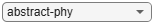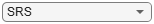Simulation Logs

The parameters used for simulation and the simulation logs are saved in MAT-files for post-simulation analysis and visualization. The simulation parameters are saved in a MAT-file with the file name as the value of configuration parameter parametersLogFile. For more details, see the NR Intercell Interference Modeling example.

if enableTraces
% Get the logs
simulationLogs = cell(numSitesPerCluster, 1);
for siteIdx = 1:numCellsOfInterest
if(simParameters.DuplexMode == 0) % FDD
logInfo = struct('NCellID', [], 'DLTimeStepLogs', [], 'ULTimeStepLogs', [], 'SchedulingAssignmentLogs', [], 'BLERLogs', [], 'AvgBLERLogs', []);
else % TDD
logInfo = struct('TimeStepLogs', [], 'SchedulingAssignmentLogs', [], 'BLERLogs', [], 'AvgBLERLogs', []);
end
logInfo.SchedulingAssignmentLogs = getGrantLogs(simSchedulingLogger{siteIdx}); % Scheduling assignments log
end
save(parametersLogFile, 'simParameters'); % Save simulation parameters in a MAT-file
save(simulationLogFile, 'simulationLogs'); % Save simulation logs in a MAT-file
end

 3GPP TS 38.104. “NR; Base Station (BS) radio transmission and reception.” 3rd Generation Partnership Project; Technical Specification Group Radio Access Network.

 3GPP TS 38.214. “NR; Physical layer procedures for data.” 3rd Generation Partnership Project; Technical Specification Group Radio Access Network.

 3GPP TS 38.321. “NR; Medium Access Control (MAC) protocol specification.” 3rd Generation Partnership Project; Technical Specification Group Radio Access Network.

 3GPP TS 38.322. “NR; Radio Link Control (RLC) protocol specification.” 3rd Generation Partnership Project; Technical Specification Group Radio Access Network.

 3GPP TS 38.323. “NR; Packet Data Convergence Protocol (PDCP) specification.” 3rd Generation Partnership Project; Technical Specification Group Radio Access Network.

 3GPP TS 38.331. “NR; Radio Resource Control (RRC) protocol specification.” 3rd Generation Partnership Project; Technical Specification Group Radio Access Network.

 3GPP TR 37.910. “Study on self evaluation towards IMT-2020 submission.” 3rd Generation Partnership Project; Technical Specification Group Radio Access Network.